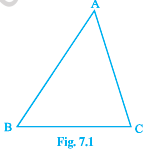# NCERT Class 9 Maths Triangles

Read and download NCERT Class 9 Maths Triangles chapter in NCERT book for Class 9 Mathematics. You can download latest NCERT eBooks chapter wise in PDF format free from Studiestoday.com. This Mathematics textbook for Class 9 is designed by NCERT and is very useful for students. Please also refer to the NCERT solutions for Class 9 Mathematics to understand the answers of the exercise questions given at the end of this chapter

## NCERT Book for Class 9 Mathematics Chapter 7 Triangles

Class 9 Mathematics students should refer to the following NCERT Book chapter Chapter 7 Triangles in standard 9. This NCERT Book for Grade 9 Mathematics will be very useful for exams and help you to score good marks

### Chapter 7 Triangles NCERT Book Class 9

You have studied about triangles and their various properties in your earlier classes. You know that a closed figure formed by three intersecting lines is called a triangle. (‘Tri’ means ‘three’). A triangle has three sides, three angles and three vertices. For example, in triangle ABC, denoted as D ABC (see Fig. 7.1); AB, BC, CA are the three sides, Ð A, Ð B, Ð C are the three angles and A, B, C are three vertices. In Chapter 6, you have also studied some properties of triangles. In this chapter, you will study in details about the congruence of triangles, rules of congruence, some more properties of triangles and inequalities in a triangle. You have already verified most of these properties in earlier classes. We will now prove some of them.7.2 Congruence of Triangles
You must have observed that two copies of your photographs of the same size are identical. Similarly, two bangles of the same size, two ATM cards issued by the same bank are identical. You may recall that on placing a one rupee coin on another minted in the same year, they cover each other completely.

Do you remember what such figures are called? Indeed they are called congruent figures (‘congruent’ means equal in all respects or figures whose shapes and sizes are both the same). Now, draw two circles of the same radius and place one on the other. What do you observe? They cover each other completely and we call them as congruent circles.

Please refer to attached file for NCERT Class 9 Maths Triangles

## Books recommended by teachers

 NCERT Class 9 Maths Number Systems NCERT Class 9 Maths Number Systems Questions
 NCERT Class 9 Maths Polynomials NCERT Class 9 Maths Polynomials Questions
 NCERT Class 9 Maths Coordinate Geometry NCERT Class 9 Maths Coordinate Geometry Questions
 NCERT Class 9 Maths Linear Equations In Two Variables NCERT Class 9 Maths Linear Equations In Two Variables Questions
 NCERT Class 9 Maths Introduction To Euclids Geometry NCERT Class 9 Maths Introduction To Euclids Geometry Questions
 NCERT Class 9 Maths Lines And Angles NCERT Class 9 Maths Lines And Angles Questions
 NCERT Class 9 Maths Triangles NCERT Class 9 Maths Triangles Questions